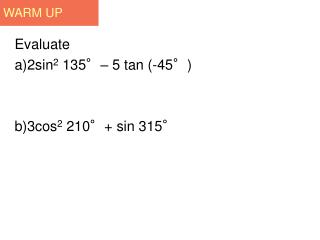DownloadDownload PresentationWARM UP

# WARM UP

Télécharger la présentation## WARM UP

- - - - - - - - - - - - - - - - - - - - - - - - - - - E N D - - - - - - - - - - - - - - - - - - - - - - - - - - -
##### Presentation Transcript

1. WARM UP Evaluate • 2sin2 135°– 5 tan (-45°) • 3cos2 210°+ sin 315°

2. Trig Game Plan Date: 9/30/13

3. 2 Acute Angles and Right Triangle

4. Caution When evaluating trigonometric functions of angles given in degrees, remember that the calculator must be set in degree mode.

5. Example 1 FINDING FUNCTION VALUES WITH A CALCULATOR Approximate the value of each expression. (a) sin 49°12′ ≈ .75699506 (b) sec 97.977° Calculators do not have a secant key, so first find cos 97.977° and then take the reciprocal. sec 97.977° ≈ –7.205879213

6. Example 1 Use the reciprocal identity FINDING FUNCTION VALUES WITH A CALCULATOR (continued) Approximate the value of each expression. (c) ≈ .91354546 (d) sin (–246°)

7. 2.3Example 2 Finding Function Values with a Calculator (page 67) Approximate the value of each expression.

8. Angle Measures Using a Calculator • Graphing calculators have three inverse functions. • If x is an appropriate number, then gives the measure of an angle whose sine, cosine, or tangent is x.

9. SUMMARY

10. Example 3: Using Inverse Trigonometric Functions to Find Angles • Use a calculator to find an angle in the interval that satisfies each condition. Using the degree mode and the inverse sine function, we find that an angle having sine value .8535508 is 58.6 . We write the result as

11. Example 3: Using Inverse Trigonometric Functions to Find Angles continued Use the identity Find the reciprocal of 2.48679 to get Now find using the inverse cosine function. The result is 66.289824

12. USING INVERSE TRIGONOMETRIC FUNCTIONS TO FIND ANGLES Example 4 Use the identity Use a calculator to find an angle θ in the interval [0°, 90°] that satisfies each condition. (a) Use degree mode and the inverse sine function. (b)

13. Caution Note that the reciprocal is used before the inverse trigonometric function key when finding the angle, but after the trigonometric function key when finding the trigonometric function value.

14. Example 5a Use a calculator to find an angle θ in the interval[0°, 90°] that satisfies each condition.

15. Example 5b

16. Example 5c

17. Time of Duty (TOD) Explain in at least three sentences to use a calculator to find an angle β in the interval [0°, 90°] that satisfies cot β ≈ 1.5789.